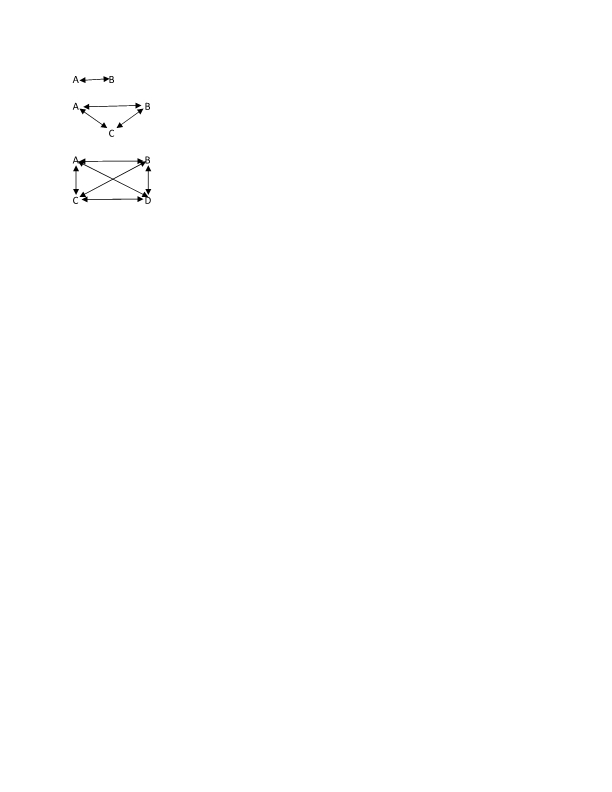# Neil Cole#### Group Dynamics

Tucked in a 64 year old book is a mathematical formula that gives clues about grouping procedures. Bossard1 points out that the number of people in a group may increase by simple mathematical progression, but the increase of relationships comes through geometric progression. Two variables are defined. Let Y equal the number of persons in…